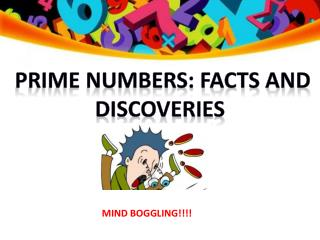Download Presentationprime numbers: facts and discoveries

# prime numbers: facts and discoveries - PowerPoint PPT PresentationDownload Presentation## prime numbers: facts and discoveries

- - - - - - - - - - - - - - - - - - - - - - - - - - - E N D - - - - - - - - - - - - - - - - - - - - - - - - - - -
##### Presentation Transcript

1. prime numbers: facts anddiscoveries MIND BOGGLING!!!!

2. Facts: The largest known prime number • The largest known prime number is 257,885,161 − 1, a number with 17,425,170 digits.

3. How many prime numbers are there? • The table below gives you an indication of how many prime numbers there are in all numbers. The percentage decreases with the increase in numbers

4. Are there infinite number of primes?? • A proof that there are an infinite number of primes: • Assume there is a finite number of primes. • We then multiply all these primes together. The resulting number will be a number divisible by every single prime. • Now add 1. We now have a number that, when you divide it by any prime, will have a remainder of 1. • That means we have just discovered another prime • (This proof was proposed by Euclid in his work Elements (Book IX, Proposition 20)

5. Interesting discoveries: Truncatable primes You can drop off any amount of digits from a side and the resulting number will remain prime • 73939133is the largest known right- truncatable prime. • 357686312646216567629137is the largest known left- truncatable prime.

6. Carrousel Primes • It deals with the 1379 digits in the number. You replace all the ones with threes, all the threes with sevens, all the sevens with nines, and all the nines with ones. Then the fun part is trying to find out if the transformed number is also prime. 19 -> 31 -> 73 ->97 131 -> 373 -> 797 -> 919 157 -> 359 -> 751 -> 953 821 -> 823 -> 827 -> 829 2111->2333->2777->2999

7. Heinz Rectangle puzzle • You make a rectangle of prime numbers where all the rows and the columns have prime numbers . Each number following the pervious must be the next prime number. The sums of the rows will also be prime.

8. Example:

9. Prime Number Trick • 1. Ask your friends to pick any prime number greater than 3.2. Square it.3. Add 14.4. Divide by 12. • Without knowing which prime number your friends picked, you can still tell them: There will be a remainder of 3.

10. The mathematics behind this is rather simple.1. Let p be a prime number, p > 3.2. Squaring gives:     p^2.3. Adding 14 gives:     p^2 + 144. Taking it modulo 12 gives:    (p^2 + 14) mod 12 We want to show that:    (p^2 + 14) mod 12 = 3This is equivalent to:    p^2 – 1 is divisible by 12.That is:    (p-1)(p+1) is divisible by 12.

11. For a number to be divisible by twelve, it has to be divisible both by 3 and by 4. We know that, out of p-1, p and p+1, one of them must bedivisible by 3; and it can’t be p, because p is prime and greater than 3. Thus, either p-1 or p+1 is divisible by 3, and so their product is also:    (p-1)(p+1) is divisible by 3. Now, since p is a prime greater than 3, we know that it is odd. Therefore, both p-1 and p+1 are even numbers. The product of two even numbers is divisible by 4, so:    (p-1)(p+1) is divisible by 4. Combining this with the above, we get that:    (p-1)(p+1) is divisible by 12.And hence:    (p^2 + 14) mod 12 = 3

12. Despite their importance, the distribution of prime numbers among the integers is still a mystery. • There is no pattern dictating which numbers will be prime or how far apart successive primes will be. • The seeming randomness of the primes makes the pattern found in "Ulam spirals" very strange indeed. • In 1963, the mathematician Stanislaw Ulam noticed an odd pattern while doodling in his notebook during a presentation: When integers are written in a spiral, prime numbers always seem to fall along diagonal lines Prime spiral

13. A VERY INTERESTING FACT ABOUT THE NUMBER 37: OBSERVATIONS: • All the three digit numbers on the left hand side are divisible by 37. • All the numbers on the right hand side are divisible by the number 3. • 111 / 37 = 3 • 222 / 37 = 6 • 333 / 37 = 9 • 444 / 37 = 12 • 555 / 37 = 15 • 666 / 37 = 18 • 777 / 37 = 21 • 888 / 37 = 24 • 999 / 37 = 27

14. AN INTERESTING USE Prime numbers are not all that useful but they are interesting. Here is an interesting fact that you may like to think about. The astronomer Carl Sagan wanted to broadcast prime numbers to the Universe as a signal to alien life forms that we exist & are intelligent.

15. bibliography www.google.com www.wikipedia.com www.livescience.com/26584-5-mind-boggling-math-facts.html www.murderousmaths.co.uk

16. Efforts by: GROUP 4 Ritu Samiksha Shagun Somali